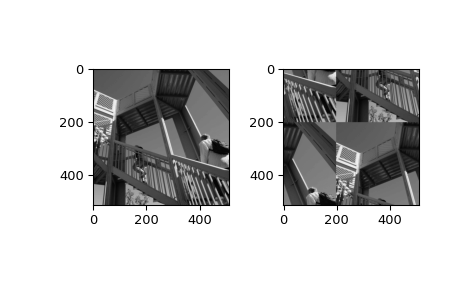# scipy.ndimage.fourier_shift¶

scipy.ndimage.fourier_shift(input, shift, n=- 1, axis=- 1, output=None)[source]

Multidimensional Fourier shift filter.

The array is multiplied with the Fourier transform of a shift operation.

Parameters
inputarray_like

The input array.

shiftfloat or sequence

The size of the box used for filtering. If a float, shift is the same for all axes. If a sequence, shift has to contain one value for each axis.

nint, optional

If n is negative (default), then the input is assumed to be the result of a complex fft. If n is larger than or equal to zero, the input is assumed to be the result of a real fft, and n gives the length of the array before transformation along the real transform direction.

axisint, optional

The axis of the real transform.

outputndarray, optional

If given, the result of shifting the input is placed in this array. None is returned in this case.

Returns
fourier_shiftndarray

The shifted input.

Examples

>>> from scipy import ndimage, misc
>>> import matplotlib.pyplot as plt
>>> import numpy.fft
>>> fig, (ax1, ax2) = plt.subplots(1, 2)
>>> plt.gray()  # show the filtered result in grayscale
>>> ascent = misc.ascent()
>>> input_ = numpy.fft.fft2(ascent)
>>> result = ndimage.fourier_shift(input_, shift=200)
>>> result = numpy.fft.ifft2(result)
>>> ax1.imshow(ascent)
>>> ax2.imshow(result.real)  # the imaginary part is an artifact
>>> plt.show()#### Previous topic

scipy.ndimage.fourier_gaussian

#### Next topic

scipy.ndimage.fourier_uniform